# Inductance Formula Circuits – Series and Parallel InductorsIf you have read the previous post about what is an inductor, let us proceed to the next level, what is the inductance formula circuits.

Contents

Now that the inductor has been added to our list of passive elements, it is necessary to extend the powerful tool of series-parallel combination. We need to know how to find the equivalent inductance of a series-connected or parallel-connected set of inductors found in practical circuits.

## Series Inductors

Consider a series connection of N inductors, as shown in Figure.(1a), with the equivalent circuit shown in Figure.(1b).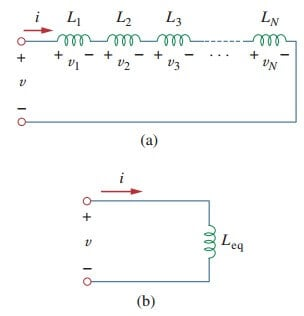Figure 1. (a) A series connection of N inductors, (b) equivalent circuit for the series inductors.

The inductors have the same current through them. Applying KVL to the loop,

Substituting vk​=​ Lk di/dt results in

where

Thus,

The equivalent inductance of series-connected inductors is the sum of the individual inductances.

Inductors in series are combined in exactly the same way as resistors

## Parallel Inductors

We now consider a parallel connection of N inductors, as shown in Figure.(2a), with the equivalent circuit in Figure.(2b). The inductors have the same voltage across them. Using KCL,

But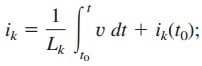hence,

where

The initial current i(t0) through Leq at t​=​ t0 is expected by KCL to be the sum of the inductor currents at t0. Thus, according to Equation.(5),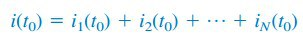According to Equation.(6),

The equivalent inductance of parallel inductors is the reciprocal of the sum of the reciprocals of the individual inductances.

Note that the inductors in parallel are combined in the same way as resistors in parallel.

For two inductors in parallel (N = 2), Equation.(6) becomes

## Inductance Formula Circuits Examples

1. Find the equivalent inductance of the circuit shown in Figure.(3).

Solution:
The 10-H, 12-H, and 20-H inductors are in series; thus, combining them gives a 42-H inductance. This 42-H inductor is in parallel with the 7-H inductor so that they are combined, to give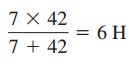This 6-H inductor is in series with the 4-H and 8-H inductors. Hence,2. For the circuit in Figure.(4),
i(t) = 4(2 − e−10t) mA. If i2(0) = −1 mA, find:
(a) i1(0);
(b) v(t), v1(t), and v2(t);
(c) i1(t) and i2(t).

Solution:
(a) From i(t) = 4(2 − e−10t) mA, i(0) = 4(2 − 1) = 4 mA.
Since i = i1 + i2,(b) The equivalent inductance isThus,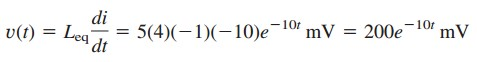andSince v = v1 + v2,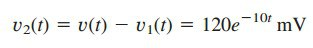(c) The current i1 is obtained asSimilarly,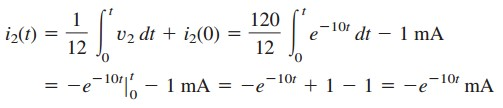Note that i1(t) + i2(t) = i(t).